## Setup

We use the iris dataset, and ampute the data:

require(miceRanger)
set.seed(1)

data(iris)

# Ampute the data. iris contains no missing values by default.
ampIris <- amputeData(iris,perc=0.25)

miceObj <- miceRanger(
ampIris
, m=6
, verbose=FALSE
)

## Diagnostic Plotting

miceRanger comes with an array of diagnostic plots that tell you how valid the imputations may be, how they are distributed, which variables were used to impute other variables, and so on.

### Distribution of Imputed Values

We can take a look at the imputed distributions compared to the original distribution for each variable:

plotDistributions(miceObj,vars='allNumeric')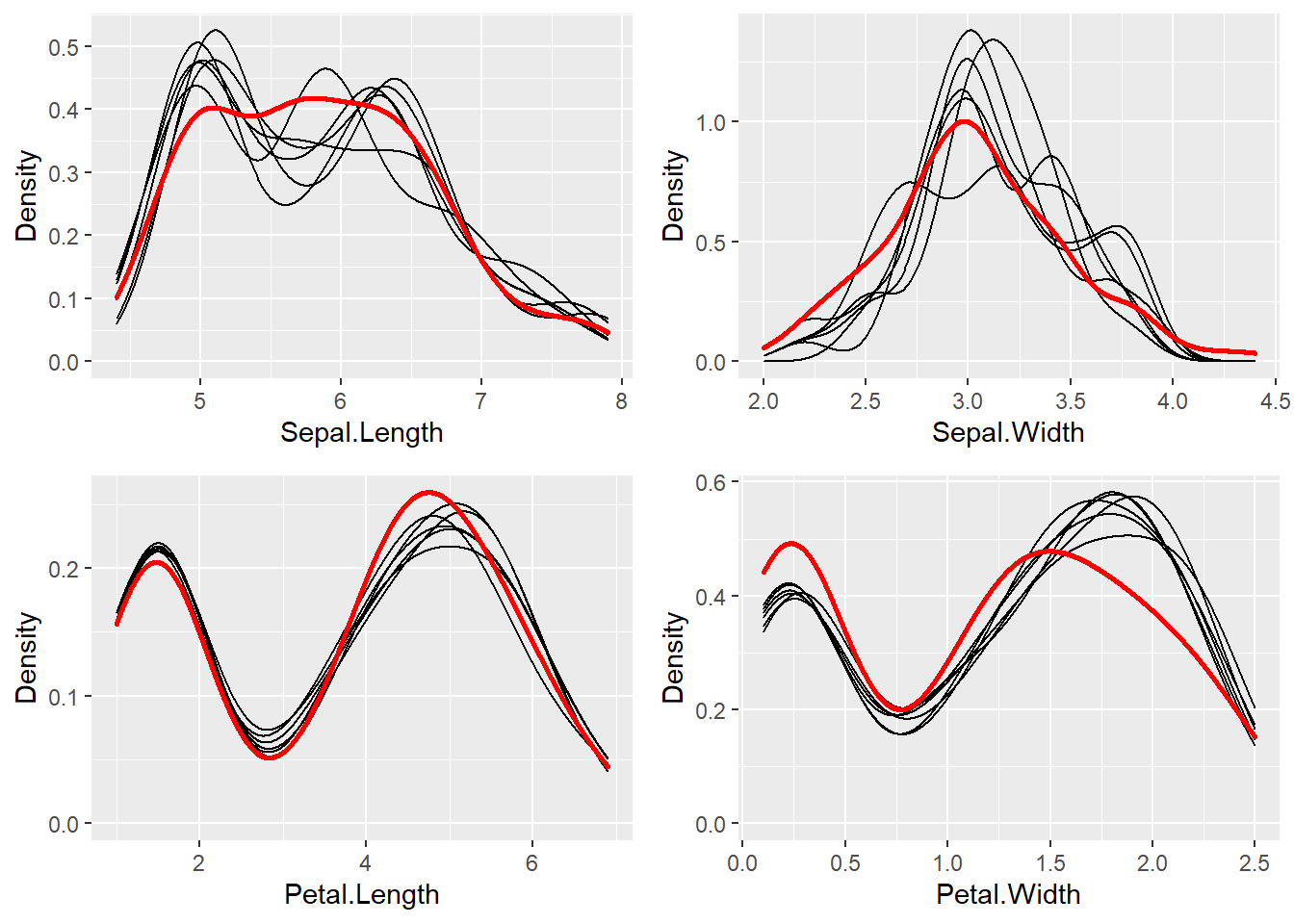The red line is the density of the original, nonmissing data. The smaller, black lines are the density of the imputed values in each of the datasets. If these don’t match up, it’s not a problem, however it may tell you that your data was not Missing Completely at Random (MCAR).

### Convergence of Correlation

We are probably interested in knowing how our values between datasets converged over the iterations. The plotCorrelations function shows you a boxplot of the correlations between imputed values in every combination of datasets, at each iteration:

plotCorrelations(miceObj,vars='allNumeric')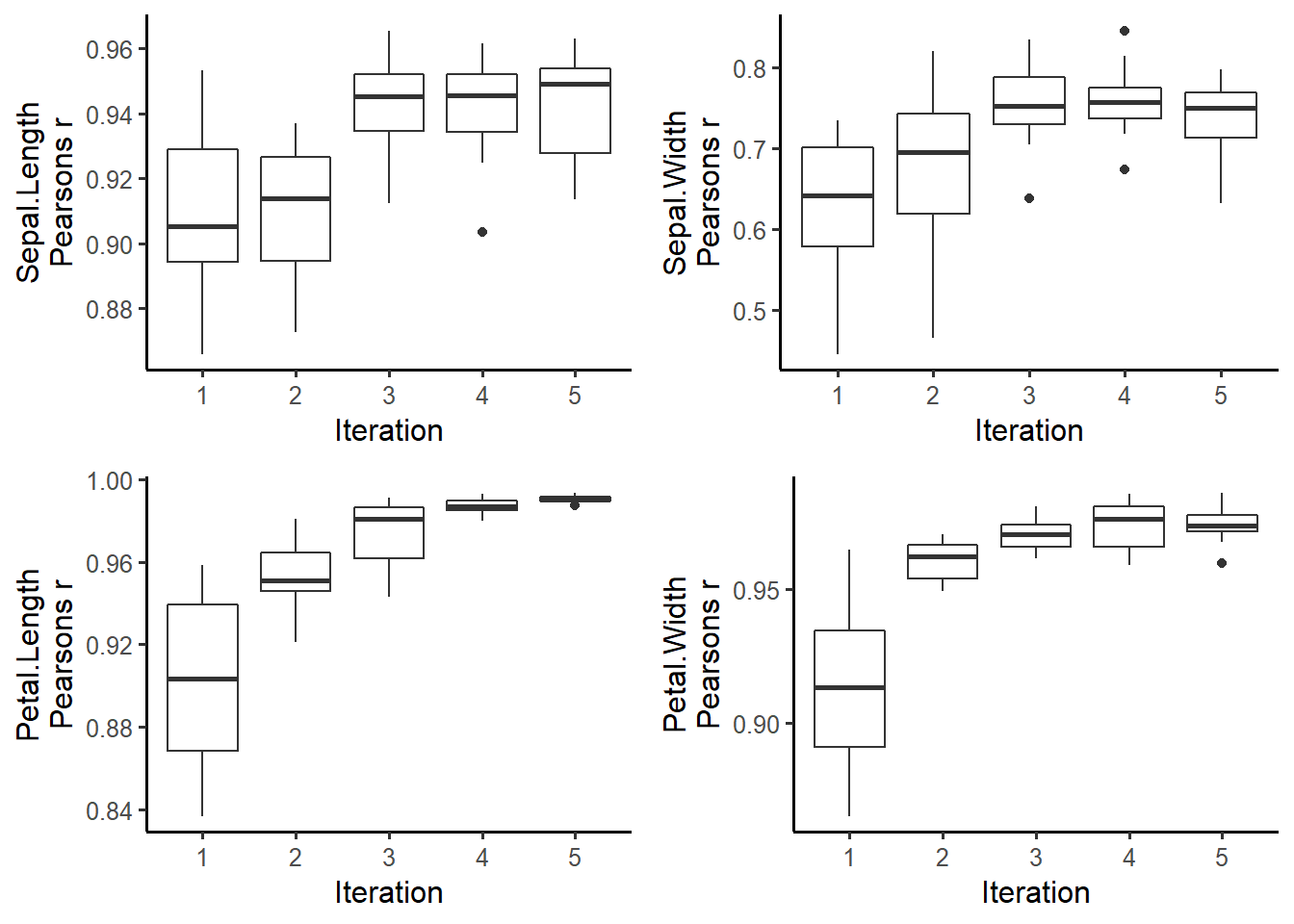Different correlation measures can be plotted by specifying factCorrMetric and numbCorrMetric. See ?plotCorrelations for more details.

### Center and Dispersion Convergence

Sometimes, if the missing data locations are correlated with higher or lower values, we need to run multiple iterations for the process to converge to the true theoretical mean (given the information that exists in the dataset). We can see if the imputed data converged, or if we need to run more iterations:

plotVarConvergence(miceObj,vars='allNumeric')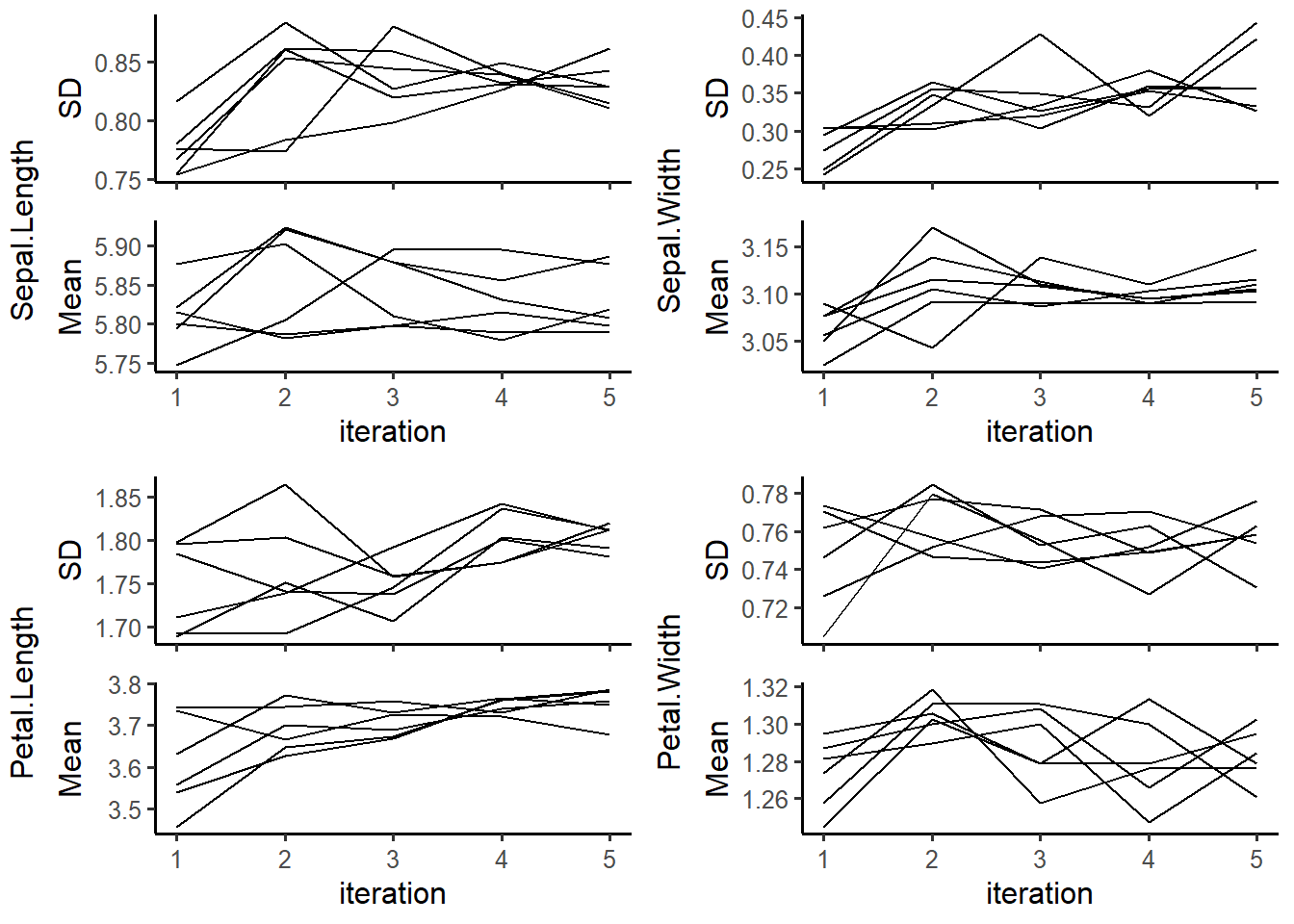It doesn’t look like this dataset had a convergence issue. We wouldn’t expect one, since we amputed the data above completely at random for each variable. When plotting categorical variables, the center and dispersion metrics plotted are the percent of the mode and the entropy, respectively.

### Model OOB Error

Random Forests give us a cheap way to determine model error without cross validation. Each model returns the OOB accuracy for classification, and r-squared for regression. We can see how these converged as the iterations progress:

plotModelError(miceObj,vars='allNumeric')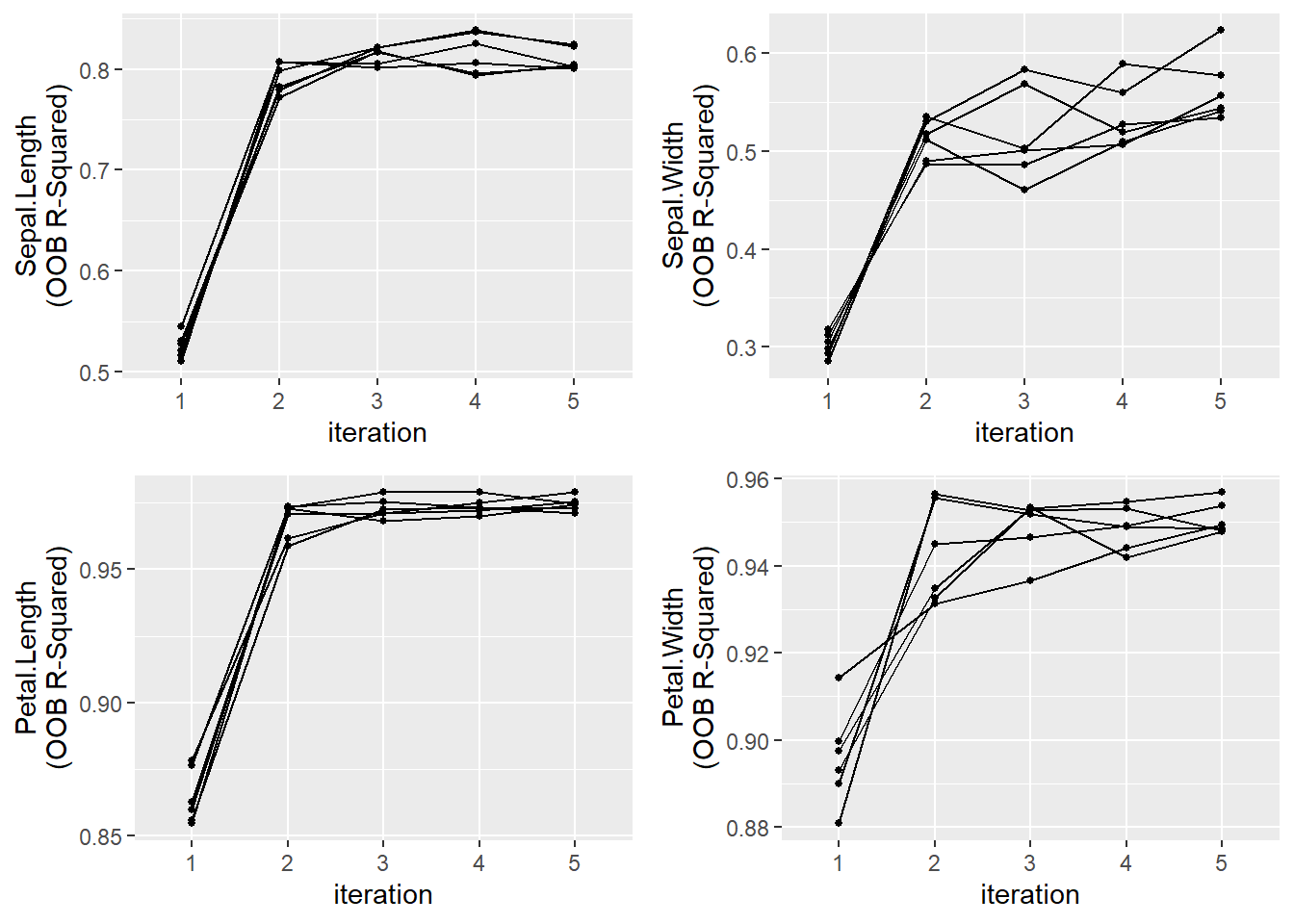It looks like the variables were imputed with a reasonable degree of accuracy. That spike after the first iteration was due to the nature of how the missing values are filled in before the models are run.

### Variable Importance

Now let’s plot the variable importance for each imputed variable. The top axis contains the variable that was used to impute the variable on the left axis.

plotVarImportance(miceObj)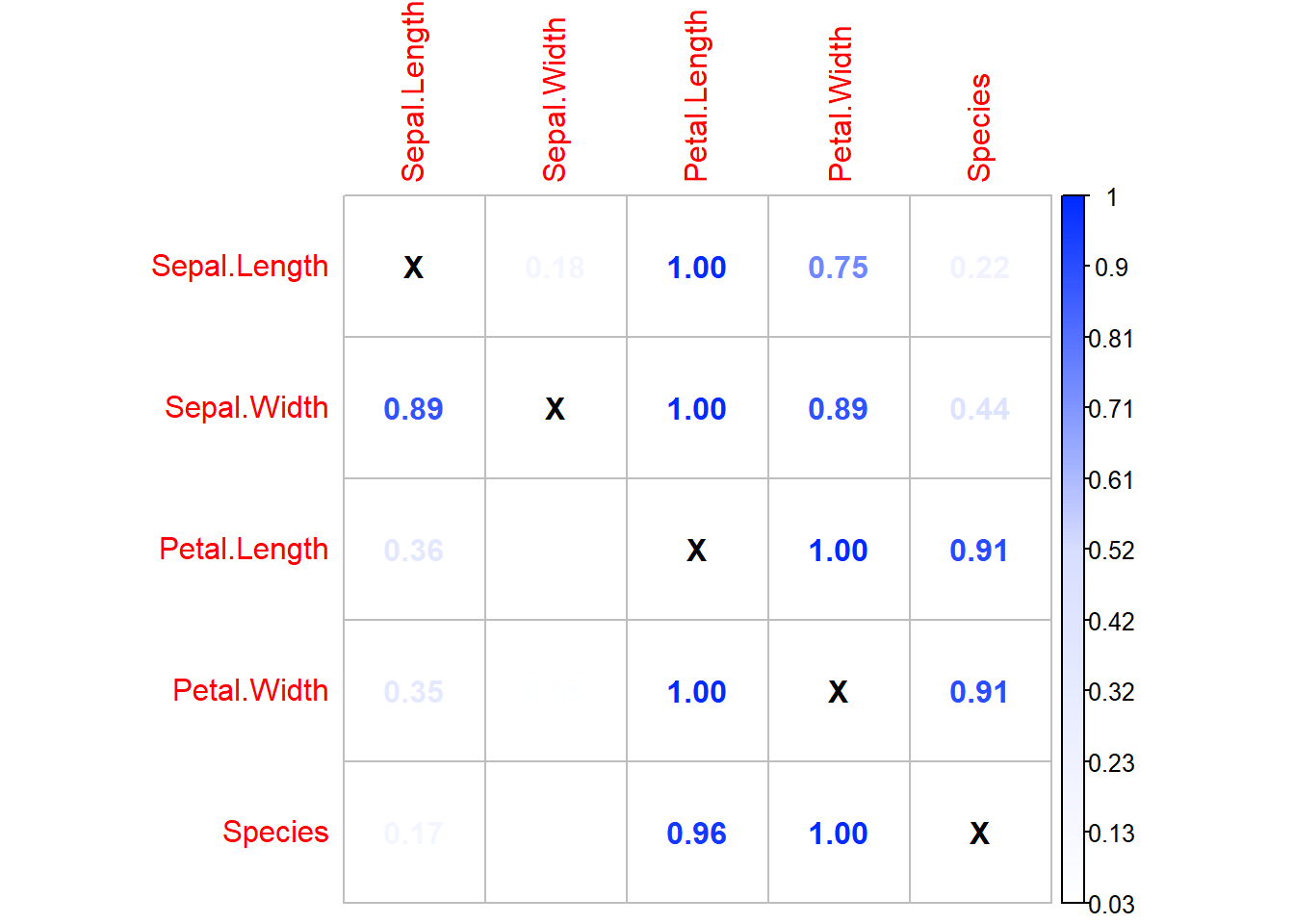The variable importance metric used is returned by ranger when importance = 'impurity'. Due to large possible variances in the returned value, the data plotted here has been 0-1 scaled within each imputed variable. Use display = 'Absolute' to show unscaled variable importance.

### Imputed Variance Between Datasets

We are probably interested in how “certain” we were of our imputations. We can get a feel for the variance experienced for each imputed value between the datasets by using plotImputationVariance() function:

plotImputationVariance(miceObj,ncol=2,widths=c(5,3))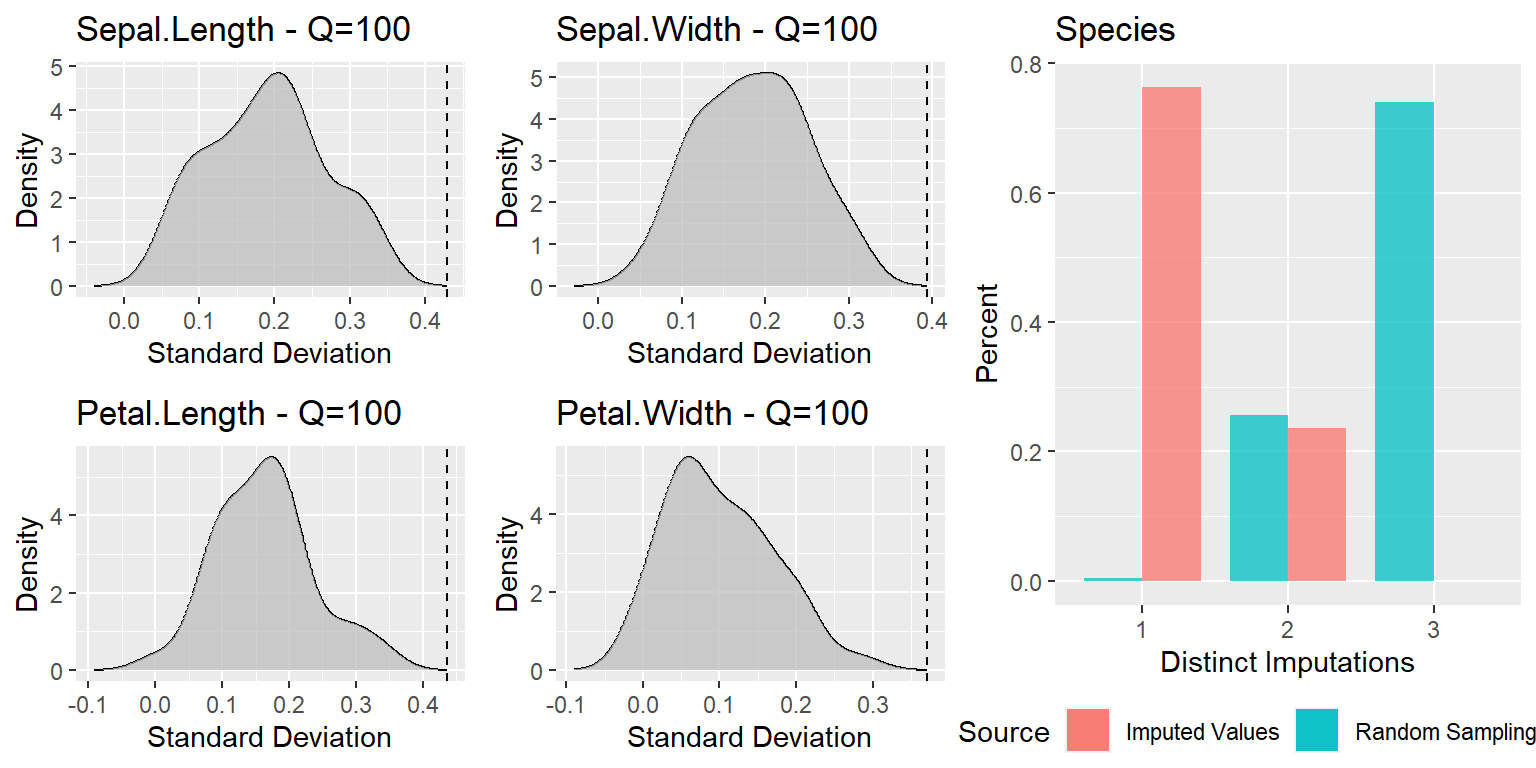When plotting categorical data, the distribution of the number of unique imputed levels is compared to the theoretical distribution of unique levels, given they were drawn randomly. You can see that most of the imputed values only had 1 imputed value across our 8 datasets, which means that the imputation process was fairly ‘certain’ of that imputed class. According to the graph, most of our samples would have had 3 different samples drawn, if they were drawn randomly for each dataset sample.
When plotting the variance of numeric features, the standard deviation of the imputed values is calculated for each sample. This is then compared to the total population standard deviation. Percentage of the samples with a SD below the population SD is shaded in the densities above, and the Quantile is shown in the title. The iris dataset tends to be full of correlation, so all of our imputations had a SD lower than the population SD, however this will not always be the case.